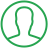# How To Find Data Type In Python

Last Updated : Jul 1, 2023In this article we will show you the solution of how to find data type in python, Python's data type can be determined by displaying the data type of the data. The type() function in Python allows you to determine what data type data is.

Once a variable has been passed as a parameter, Type() returns its data type.

The type() function in Python lets you find out what data type is being used like Strings, ints, floats, lists, and tuples are among the available data types.

The type() function is very useful when debugging an error because it can tell you the data type of the error.

As an example, if you are doing a mathematical operation, strings cannot be added to integers or floats.

When you try to add a string to a float, for example, Python will throw an error.

You can determine what data type each variable is by using the type() function on each variable. determining the type of data is very important when using the type() function.

The Python language uses datatypes to identify variables. Unlike Java or C++, Python doesn't require type definitions. Datatypes must be declared during the declaration phase of most programming languages, while Python has explicit datatype conversion (which automatically takes the right datatype based on the context).

We will now discuss the idea of a how to find data type in python with example.

## Step By Step Guide On How To Find Data Type In Python :-

```str_list = "Welcome to TalkersCode"
age = 50
pi = 3.14
c_num = 3j+10
my_list = ["X", "Y", "Z"]
my_tuple = ("X", "Y", "Z")
my_dict = {"X":"x", "Y":"y", "Z":"z"}
my_set = {'X', 'Y', 'Z'}
print("The type is : ",type(str_list))
print("The type is : ",type(age))
print("The type is : ",type(pi))
print("The type is : ",type(c_num))
print("The type is : ",type(my_list))
print("The type is : ",type(my_tuple))```
1. Define a string variable named "str_list" with the value "Welcome to TalkersCode".
2. Define an integer variable named "age" with the value 50.
3. Define a float variable named "pi" with the value 3.14.
4. Define a complex number variable named "c_num" with the value 3j+10.
5. Define a list variable named "my_list" with the values ["X", "Y", "Z"].
6. Define a tuple variable named "my_tuple" with the values ("X", "Y", "Z").
7. Define a dictionary variable named "my_dict" with the key-value pairs {"X":"x", "Y":"y", "Z":"z"}.
8. Define a set variable named "my_set" with the values {'X', 'Y', 'Z'}.
9. Then Print the type of "str_list".
10. Then Print the type of "age".
11. After that Print the type of "pi".
12. Then Print the type of "c_num".
13. Then Print the type of "my_list".
14. After that Print the type of "my_tuple".

## Conclusion :-

As a result, we have successfully learned how to find data type in python.

A value can be checked for a specific data type using the Python isinstance() function, whereas its exact data type can be determined by type().

Due to the fact that it only has one parameter, this method is advantageous over the others.

Furthermore, the isinstance() function is not efficient when it comes to debugging.

When a programmer needs to test data types or debug an issue, the isinstance() function is a great alternative. Alternatively, type() can also be used as a Boolean operation or result.

I hope this article on how to find data type in python helps you and the steps and method mentioned above are easy to follow and implement.

##About Riya

A recent graduate with a Bachelor of Technology (B.Tech) in Computer Science from India. She is passionate about leveraging technology to solve real-world problems. With a strong foundation and experience in programming languages such as Python, Django, HTML, CSS, and JavaScript, java, php and have honed her skills through hands-on projects and coursework.